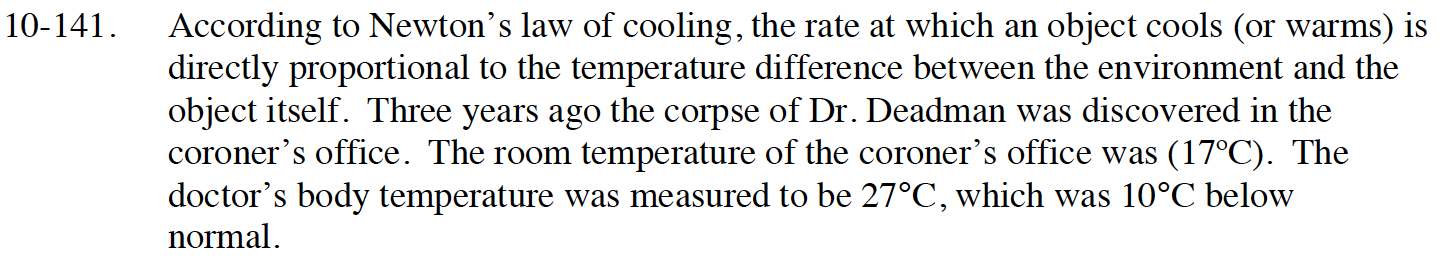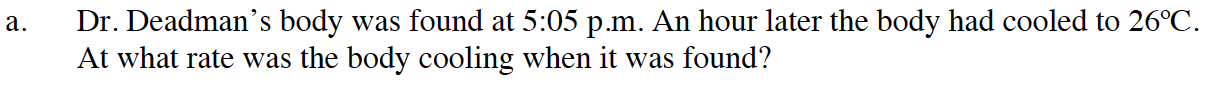### Home > CALC > Chapter 10 > Lesson 10.4.1 > Problem10-141

10-141.$\frac{dT}{dt}=-k(T-17)$

After solving the differential equation:

$T(t)=Ce^{-kt}+17$

Now use (0, 27) and (1, 26) to solve for the values of the parameters.

What is T′(0)?Normal body temperature is 37ºC.